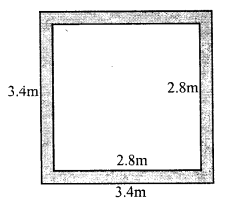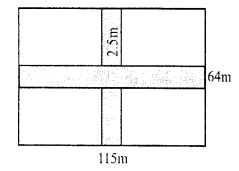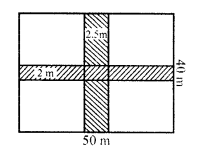# RS Aggarwal Class 7 Solutions Chapter 20 Mensuration Ex 20B

In this chapter, we provide RS Aggarwal Solutions for Class 7 Chapter 20 Mensuration Ex 20B for English medium students, Which will very helpful for every student in their exams. Students can download the latest RS Aggarwal Solutions for Class 7 Chapter 20 Mensuration Ex 20B Maths pdf, free RS Aggarwal Solutions Class 7 Chapter 20 Mensuration Ex 20B Maths book pdf download. Now you will get step by step solution to each question.

### RS Aggarwal Solutions for Class 7 Chapter 20 Mensuration Ex 20B Download PDF

Question 1.
Solution:
Outer length of plot (L) = 75 m
and breadth (B) = 60 m
Width of path inside = 2 mInner length (l) = 75 – 2 x 2 = 75 – 4 = 71 m
and width (b) = 60 – 2 x 2 = 60 – 4 = 56 m
Area of the path = L x B – l x b = (75 x 60 – 71 x 56) m² = 4500 – 3976 = 524 m²
Rate of constructing it = Rs. 125 per m²
Total cost = Rs. 524 x 125 = Rs. 65500

Question 2.
Solution:
Outer length of the plot (L) = 95 m
and breadth (B) = 72 m
Width of path = 3.5 mInner length (l) = 95 – 2 x 3.5 = 95 – 7 = 88 m
and breadth = 72 – 2 x 3.5 = 72 – 7 = 65 m
Outer area = L x B = 95 x 72 m² = 6840 m²
and inner area = l x b = 88 x 65 m² = 5720 m²
Area of path = outer area – inner area = 6840 – 5720 = 1120 m²
Rate of constructing it = Rs. 80 per m²
Total cost = Rs. 1120 x 80 = Rs. 89600
and rate of laying grass = Rs. 40 per m²
Total cost = Rs. 40 x 5720 = Rs. 228800
Total cost = Rs. 89600 + Rs. 228800 = Rs. 318400

Question 3.
Solution:
Length of saree (L) = 5 m
and breadth (B) = 1.3 m
Width of border = 25 cm
Inner length (l) = 5 – 2×25100 = 5 – 0.5 = 4.5 m
and inner breadth (b) = 1.3 – 2×25100 = 1.3 – 0.5 = 0.8 m
Now area of the boarder = L x B – l x b
= (5 x 1.3 – 4.5 x 0.8) m²
= 6.50 – 3.60
= 2.90 m²
= 2.90 x 100 x 100 = 29000 cm²
Cost of 10 cm² = Re. 1
Total cost = Rs. 29000×110 = Rs. 2900

Question 4.
Solution:
Inner length of lawn (l) = 38 m
and breadth (b) = 25 m.
Width of path = 2.5 m.
Outer length (L) = 38 + 2 x 2.5 = 38 + 5 = 43 m
and outer breadth (B) = 25 + 2 x 2.5 = 25 + 5 = 30 m
Area of path = Outer area – Inner area
= (43 x 30 – 38 x 25) m²
=(1290 – 950) m² = 340 m²
Rate of gravelling the path = Rs. 120 per m²
Total cost = Rs. 120 x 340 = Rs. 40800

Question 5.
Solution:
Length’ of room (l) = 9.5m
Width of outer verandah = 1.25m
Outer length (L) = 9.5 + 2 x 1.25 = 9.5 + 2.5 = 12.0 m
and breadth (B) = 6 + 2 x 1.25 = 6 + 2.5 = 8.5 m
Area of verandah = Outer area – Inner area = L x B – l x b
= (12.0 x 8.5 – 9.5 x 6) m² – (102.0 – 57.0) m² = 45 m²
Rate of cementing = Rs. 15 per m²
Total cost = Rs. 80 x 45 = Rs. 3600

Question 6.
Solution:
Each side of square bed (a) = 2m 80 cm = 2.8 m
Width of strip = 30cm
Outer side (A) = 2.8 m + 2 x 30cm = 2.8 + 0.6 = 3.4m
Outer area = (3.4 m)² = 11.56 m²Inner area = (2.8)² = 7.84 m²
Area of increased bed flower = 11.56 – 7.84 = 3.72 m²

Question 7.
Solution:
Ratio in length and breadth of the park = 2 : 1
Its perimeter = 240 m
Let length = 2x
Perimeter = 2 (2x + x) = 2 x 3x = 6x
6x = 240
⇒ x = 2406 = 40
Length = 2x = 2 x 40 = 80m
and breadth = x = 40m
Area = L x B = 80 x 40 m² = 3200 m²
Width of path inside the park = 2m
Inner length (l) = 80 – 2 x 2 = 80 – 4 = 76 m
and breadth (b) = 40 – 2 x 2 = 40 – 4 = 36 m
Inner area = 76 x 36 = 2736 m²
Area of path = Outer area – Inner area = 3200 – 2736 = 464 m²
Rate of paving the path = Rs. 80 per m²
Total cost = Rs. 80 x 464 = Rs. 37120

Question 8.
Solution:
Length of hall (l) = 22m
Space left along the walls = 75m = 34 m
Inner length (l) = 22 – 2 x 34 = 20.5 m
Inner breadth (b) = 15.5 – 2 x 34 = 15.5 – 1.5 = 14m
Area of carpet = Inner area = 20.5 x 14 m² = 287 m²
Outer area = 22 x 15.5 = 341 m²
Area of strip left out = 341 – 287 = 54 m²
Width of carpet = 82 cm = 82100 m
Length of carpet = 287 ÷ 82100
= 287×10082 = 350m
Rate of carpet = Rs. 60 per metre
Total cost = Rs. 60 x 350 = Rs. 21000

Question 9.
Solution:
Area of path = 165 m²
Width of path = 2.5m.
Let side of square lawn = x m.Outer side = x + 2 x 2.5 = (x + 5) m
Area of path = (x + 5)² – x²
⇒ x² + 10x + 25 – x² = 165
⇒ 10x = 165 – 25 = 140
⇒ x = 14010 = 14m
Side of lawn = 14m
and area of lawn = (14)² m² = 196 m²

Question 10.
Solution:
Ratio in length and breadth of a park = 5 : 2
Width of path outside it = 2.5 m
Area of path = 305 m2
Let Inner length (l) = 5x
Outer length (L) = 5x + 2 x 2.5 = (5x + 5) m
Width (B) = 2x + 2 x 2.5 = (2x + 5) m
Area of path = Outer area – Inner area
⇒ (5x + 5) (2x + 5) – 5x x 2x = 305
⇒ 10x² + 10x + 25x + 25 – 10x² = 305
⇒ 35x = 305 – 25 = 280
⇒ x = 8
Length of park = 5x = 5 x 8 = 40m
and breadth = 2x = 2 x 8 = 16m
Dimensions of park = 40m by 16m

Question 11.
Solution:
Length of lawn (l) = 70 m
Width of crossing roads = 5mArea of roads = 70 x 5 + 50 x 5 – (5)²
= 350 + 250 – (5)²
= 600 – 25 = 575 m²
Cost of constructing = Rs. 120 per m²
Total cost Rs. 120 x 575 = Rs. 69000

Question 12.
Solution:
Length of lawn (l) = 115m
Width of road parallel to length = 2mArea of roads = (115 x 2 + 64 x 2.5 – 2 x 2.5) m²
= (230 + 160 – 5) m² = (390 – 5) m² = 385 m²
Cost of gravelling = Rs. 60 m²
Total cost = Rs. 60 x 385 = Rs. 23100

Question 13.
Solution:
Length of field (l) = 50 m
and breadth (b) = 40 mWidth of road parallel to length = 2 m
Area of roads = 50 x 2 + 40 x 2.5 – 2.5 x 2 = (100 + 100 – 5) m² = 195 m²
and area of remaining portion = 50 x 40 – 195 = 2000 – 195 = 1805 m²

Question 14.
Solution:
(i) Outer length = 43m
Area = 43 x 27 = 1161 m²
Inner length = 43 – 2 x 1.5 = 43 – 3 = 40m
and breadth = 27 – 2 x 1 = 27 – 2 = 25m
Inner area = 40 x 25 = 1000 m²
Area of shaded portion = 1161 – 1000 = 161 m²
(ii) Side of square (a) = 40m
Area = (a)² = 40 x 40 = 1600 m²
Area of larger road = 40 x 3 = 120 m²
and area of shorter road = 40 x 2 = 80 m²
Area of roads = (120 + 80) – 3 x 2 = 200 – 6 = 194 m²
Area of shaded portion = (1600 – 194) m² = 1406 m²

Question 15.
Solution:
(i) Outer length = 24m
Area = 24 x 19 = 456 m²
Length of unshaded portion = 24 – 4 = 20m
Area of unshaded portion = 20 x 16.5 m² = 330.0 m²
Area of shaded portion = 456 – 330 = 126 m²
(ii) Dividing the figure an shown
Area of rectangle I = 15 x 3 cm² = 45 cm²
Area of rectangle II = (12 – 3) x 3 = 9 x 3 = 27 cm²
Area of rectangle III = 5 x 3 = 15 cm²and area of rectangle IV = (12 – 3) x 3 = 9 x 3 = 27 cm²
Total area of shaded portion = 45 + 27 + 15 + 27 = 114 cm²

Question 16.
Solution:
Dividing the figure an shown
Area of rectangle I = 3.5 x 0.5 m² = 1.75 m²
Area of rectangle II = (3.5 – 2 x 0.5) x 0.5 = (3.5 – 1) x 0.5 = 2.5 x 0.5 = 1.25 m²
Area of rectangle III = (2.5 – 1) x 0.5 = 1.5 x 0.5 = 0.75 m²
Area of rectangle IV = (1.5 – 1.0) x 0.5 x 0.5 = 0.25 m²Total area of shaded portion = (1.75 + 1.25 + 0.75 + 0.25) m² = 4 m²

All Chapter RS Aggarwal Solutions For Class 7 Maths

—————————————————————————–

All Subject NCERT Exemplar Problems Solutions For Class 7

All Subject NCERT Solutions For Class 7

*************************************************

I think you got complete solutions for this chapter. If You have any queries regarding this chapter, please comment on the below section our subject teacher will answer you. We tried our best to give complete solutions so you got good marks in your exam.

If these solutions have helped you, you can also share rsaggarwalsolutions.in to your friends.